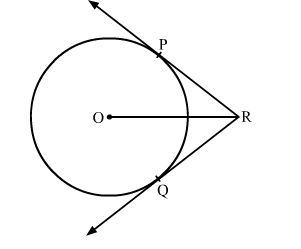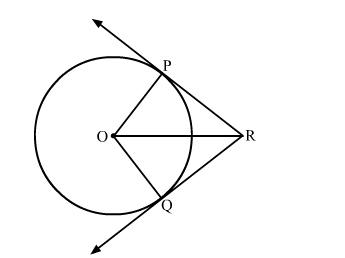# In the given figure, two tangents RQ and RP are drawn from an external point R to the circle with centre O.`
Question:

In the given figure, two tangents RQ and RP are drawn from an external point R to the circle with centre O. If ∠PRQ = 120 then prove that OR = PR + RQSolution:Construction: Join PO and OQ
In △POR and △QOR
RP = RQ    (Tangents from the external point are congruent)
OR = OR    (Common)
By SSS congruency, △POR ≅ △QOR
∠PRO = ∠QRO    (C.P.C.T)
Now, ∠PRO + ∠QRO = ∠PRQ
⇒ 2∠PRO = 120
⇒ ∠PRO = 60
Now, In △POR

$\cos 60^{\circ}=\frac{\mathrm{PR}}{\mathrm{OR}}$

$\Rightarrow \frac{1}{2}=\frac{\mathrm{PR}}{\mathrm{OR}}$

$\Rightarrow \mathrm{OR}=2 \mathrm{PR}$

$\Rightarrow \mathrm{OR}=\mathrm{PR}+\mathrm{PR}$

$\Rightarrow \mathrm{OR}=\mathrm{PR}+\mathrm{RQ}$